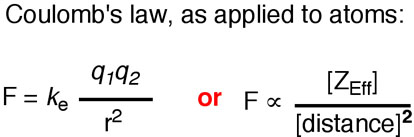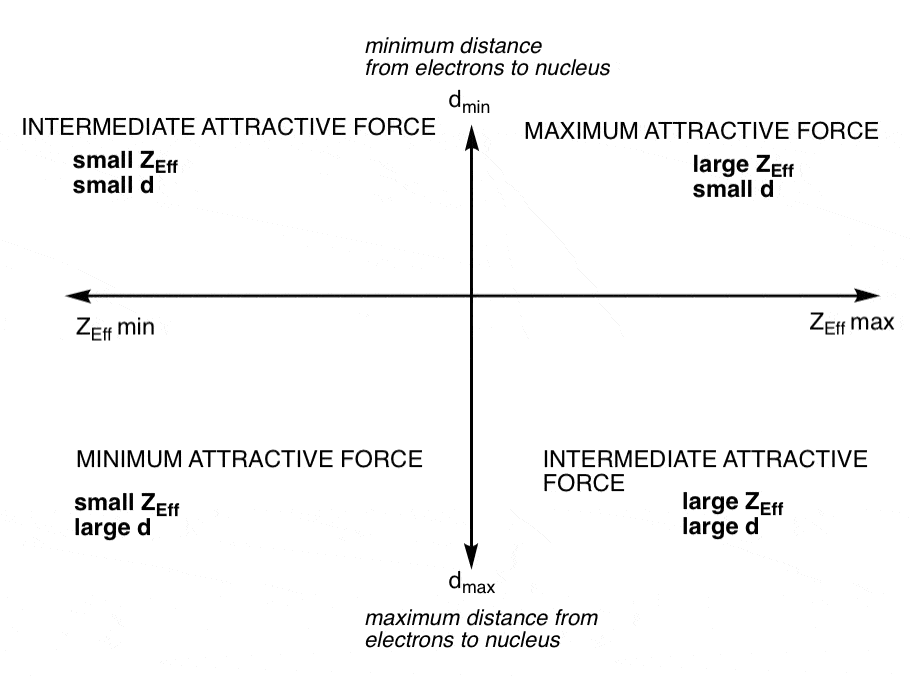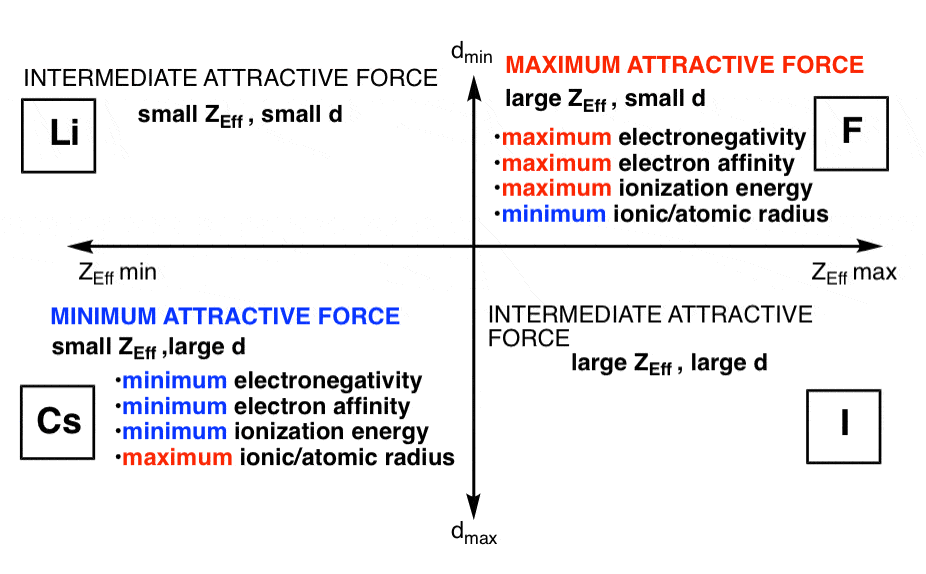# From Gen Chem to Organic Chem, Pt. 5 – Understanding Periodic Trends

Last updated: February 13th, 2020 |

Let’s revisit a few terms that you learned back in Gen chem:

1. ionization energy – the amount of energy required to remove an electron from an atom
2. electronegativity – a measure of the ability of an atom to attract electrons toward itself
3. electron affinity – the energy change when an electron is added to the neutral species to form a negative ion.
4. atomic radius – a measure of the typical distance of the atom from the nucleus to the boundary of the electron cloud
5. ionic radius – a measure of the distance across an atom’s ion in a crystal lattice.

What do these five properties have in common? They follow periodic trends.

What else do they have in common? Well, these trends can all be understood on an intuitive level by grasping the Coulomb equation.As we talked about earlier, the Coulomb equation is the equation that describes the force between two point charges. In the case of chemistry, we can use it to describe the attractive force felt between the positively charged nucleus and the negatively charged valence electrons. The magnitude of the force is determined by two things:

1. Zeff, the effective nuclear charge (the nuclear charge adjusted for shielding by the electrons between the valence electrons and the nucleus)
2. The distance d from the valence electrons to the nucleus. Like gravity, the magnitude of the force falls off proportionally to the square of the distance.  The distance from the electrons to the nucleus is largely determined by the fact that electrons inhabit a discrete set of energy levels (orbitals)  in the atom. Like attendees at an open-air concert, the seats closest to the action are taken first, while the latecomers must settle for a spot further away.

The Coulomb equation is going to be at a maximum when Zeff is big and d is small, and at a minimum when Zeff is small and d is big. Let’s make a little graph.Let’s look at the maximum and minimum cases and how they impact the terms mentioned above.

Zeff large, d small:

• The force between the valence electrons and the nucleus is at its maximum.
• electronegativity will be at a maximum
• Electron affinity will be at a maximum (assuming there is room for another electron in the orbital).
• ionization energy will also be at a maximum because the force between the nucleus and the valence electron is at its strongest.
• atomic radius and ionic radius will be at a minimum (since the Zeff is larger, the electrons will be held at a distance closer to the nucleus)

What element best fits this condition? Fluorine.

Zeff small, d large:

• The force between the valence electrons and the nucleus is at its minimum.
• Electronegativity will be at a minimum
• Electron affinity will be at a minimum
• Ionization energy will be at a minimum

What element best fits this condition? Cesium.

There’s also the two intermediate cases of low Zeff/small d and high Zeff/large d. These cases are exemplified by Lithium and Iodine respectively.

Let’s make this diagram again.Cesium and fluorine are truly the two solitudes.

The take home message: periodic trends are extremely important for understanding chemistry, and they can be grasped intuitively as a function of the attraction between the nucleus and the valence electrons.

Note: there’s another periodic trend (which I didn’t go into here) called polarizability that reflects how tightly the electrons are held by the atom. As you might expect, polarizability increases with size. It helps to explain why, for example, I(–) is a weaker conjugate base than F(–) [and hence more stable]. We’ll get to this some other time.

A final note: to get the most out of understanding periodic trends, it’s best to compare these properties either across a row or across a column – i.e. changing one variable at a time. Changing both variables at once – for instance, trying to rationalize the electronegativity of carbon versus phosphorus – can lead to variable outcomes.

Next Post – From Gen Chem to Organic Chem, Part 6 – Lewis Structures

## Comment section

### 7 thoughts on “From Gen Chem to Organic Chem, Pt. 5 – Understanding Periodic Trends”

1.T.Rathinavelu says:

I think:
“We need two charges in the numerator to use coulumb’s force equation.But there is only one charge,Zeff, in your equation.In case you are talking of “per electronic force” 1. It must be clearly mentioned.2.There may be more than one valence electron.3. ‘per charge force” is “electric field”, not force.”
T.Rathinavelu

2.Jake P says:

I am trying to get a better understanding on my homework and the first question says, “Group the electrons for each element based on ionization energies. How many electrons are in each group? You can change the scale of the vertical axis to better differentiate between groups.” I have two questions. The first one is can I use the coulomb equation to find out how many electrons are in each group? My second question is what is a “vertical axis” in your terms.

1.James says:

Thanks for the comment – sorry for late response.

I don’t think you need to use the coulomb equation.
Vertical axis is ionization energy, horizontal axis is atomic number

– not exactly sure what is being asked, but the diagram is probably something like this:
http://ths.talawanda.org/~bramblen/classroom/Pictures/first%20ion.%20graph.JPG

Use this diagram to see the patterns in ionization energies.

3.Apurv says:

James, can you help me out?
My question is more of gen Chem and inorg. I want to know the periodic trend if lattice and hydration enthalpies. That is like a question on solubility order of different compounds. I mean, why are carbonates less soluble down the group while hydroxides solubility increases (or is it the other way round?). I actually have problem only in this aspect of inorg.
You have helped me a lot in org. Org wasn’t such fun before I reached this blog. Thanks for helping out so many students like me!

1.V.Anirudh says:

Group II metal hydroxides become more soluble in water as you go down the column. This trend can be explained by the decrease in the lattice energy of the hydroxide salt and by the increase in the coordination number of the metal ion as you go down the column.
The larger the lattice energy the more energy it takes to break the lattice apart into metal and hydroxide ions. Since the atomic radii increase down the group it makes sense that the coordination numbers also increases because the larger the metal ion the more room there is for water molecules to coordinate to it.

The following examples illustrate this trend:

Magnesium hydroxide appears to be insoluble in water. However, if it is shaken in water and filtered, the solution is slightly basic. This indicates that there are more hydroxide ions in solution than there were in the original water. This is because some magnesium hydroxide has dissolved.
Calcium hydroxide solution is referred to as “lime water”. A liter of pure water will dissolve about 1 gram of calcium hydroxide at room temperature.
Barium hydroxide is soluble enough to produce a solution with a concentration around 0.1 mol dm-3 at room temperature.

But regarding the trends with carbonates,I couldn’t find any proper explantion. The solubility of carbonates DECREASES down the group.
Let me hint you that the solubility of a solid depends on many factors like lattice energy,ionization energy,hydration energy,type of solvent,etc.

I hope you find a good explantion regarding the solubility of carbonates.

4.Dhairya Patel says:

You said polarizability reflects how tightly the electrons are held together by the atom. And then you said polarizability increases with size. These sentences seems contrary to me. From what I read and understood, polarizability is the ability of an atom’s electron cloud to get distorted. And then you can say that as size increases with polarizability increases.

1.James Ashenhurst says:

The statements are not necessarily contradictory. If “polarizability” reflects how tightly the electrons are held by the atom (i.e. how easily the atom’s electron cloud can be distorted) then atoms with low polarizability (tightly held – not easily distorted electron cloud) are found at the top of the periodic table, and atoms with high polarizability (loosely held – more easily distorted electron cloud) are found near the bottom of the periodic table.

This site uses Akismet to reduce spam. Learn how your comment data is processed.